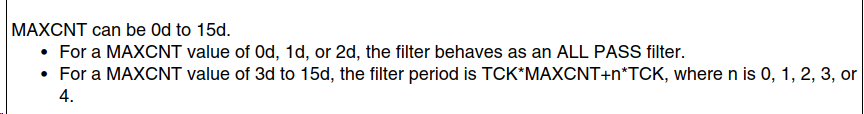# MPC57XX Glitch Filter : Minimum glitch period filter

cancel
Showing results for
Did you mean:

## MPC57XX Glitch Filter : Minimum glitch period filter

377 ViewsContributor V

As a follow-up to an earlier question about 'n' in the following equation:  [Filter Period = TCK*MAXCNTx + n*TCK]

I have calculated the minimum filter period.

Prescaled Filter Clock Period TCK = T(IRC) x (IFCP + 1)
T(IRC) = 62.5 nS (F = 16 MHz)
TCK*MAXCNTx : 62.5 * 4 = 250 nS
n*TCK: 62.5 * 4 = 250 nS
[Filter Period = TCK*MAXCNTx + n*TCK]: 250 nS + 250 nS = 500 nS

Now, I am curious to think that, at the very least, this minimal glitch filtering should be applied to all Interrupt filters regardless. Extended period filtering can be applied as necessary.

I believe this equation also determines the minimum interrupt  period. Though a interrupt at this period would take most of the processors cycles and not leave any for other tasks.

Am I correct in this assumption?

Thank you,

Labels (1)
• ### General

Tags (3)
5 Replies
247 ViewsNXP TechSupport

I am not sure if I understand your question.

The filter has nothing to do with processors cycles or task or other SW related topic.
It is just HW filter guaranting that external interrupt pulse (over external IRQ line) must withstand for certain period of time to be latched by SIU module, otherwise it is just invisible for it. It does not affect interrupt processing anyhow.

247 ViewsContributor V

Hi David,

I guess I don't understand the 'n'.

You said it was the 'uncertainty' and that it goes from 0 to 4.

The other statement reads: MAXCNTx can be 0 to 15 (for MAXCNT<3 filter will behave as all PASS filter)

So I correlated the 3 and 4 to mean that if the period is less that 4 counts/cycles, then the processor will not register the interrupt.

That being said, the very minimum period of the interrupt would be what I had calculated in the previous message.

Meaning, at the very least, that minimum filter should be standard.

Anything below that period will not register. or maybe it will due to the uncertainty?

Does that makes sense?

Thank you,

247 ViewsNXP TechSupport

There will be apparently no relation MAXCNT and 'n'. MAXCNT of 1, 2 or 3 just mean filter is disabled. MPC5744P device has is described following way (a bit better in my eyes).247 ViewsContributor V

Sorry David,

I just don't understand.

The formula is TCK*MAXCNT + n*TCK.

Now just for simplicity sake, lets assign some values. [TCK =100, MAXCNT = 5]

So if I calculate the period, the answer would be

ans1 = 100*5 + 0*100 = 500

ans2 = 100*5 + 1*100 = 600

ans3 = 100*5 + 2*100 = 700

ans4 = 100*5 + 3*100 = 800

So the period is 500, 600, 700 and 800.

or maybe the period between 500 - 800?

Thank you,

247 ViewsNXP TechSupport

You understand it right. It is just the uncertainty. Pulses shorter than 500ns definitely will be filtered, pulses longer than 800ns definitely will not be filtered. It means that in fact filter will have 500 or 600 or 700 or 800ns filtering period according to phases of both clocking domains SIU module is clocked by.